• 二维矩形装箱算法--二叉树--java实现.rarjava
• 多个车子，N个箱子，用二维矩形方式进行装车。采用二叉树实现。java
• 主要介绍了PHP实现的装箱算法,结合实例形式分析了PHP装箱算法的概念、原理、定义及使用方法,需要的朋友可以参考下PHP 装箱算法
• 装箱算法的一种应用

千次阅读 2018-10-27 13:33:59
// 装箱算法：返回金币面值总和不大于max，且差值要尽可能的小 // 这里的币值类型保证了先装大的，再装小的，不存在50，50，50，70，70，max=150这种类型的装箱 vector<int> _combcoin(vector<int>& vCount,int ...

// 1026.cpp : 定义控制台应用程序的入口点。
//

#include "stdafx.h"
#include <stdlib.h>
#include <iostream>
#include <vector>
#include <numeric>
#include <algorithm>
#include <functional>
using namespace std;

// 单次下注记录
struct stBetInfoEx
{
int index;
int coinindex;
int coinvalue;
int coincount;
int betuserid;
};

int _testIndex(int delta)
{
static int vcoinvalue={1,10,50,100,1000};
for(int i=0;i<5;i++)
{
if(delta<vcoinvalue[i])
return 2*i;
if(delta==vcoinvalue[i])
return 2*i+1;
}
return 10;
}

/*
int coin0=_testCoin(0);//0
int coin1=_testCoin(1);//1
int coin2=_testCoin(4);//1
int coin3=_testCoin(10);//10
int coin4=_testCoin(11);//10
int coin5=_testCoin(50);//50
int coin6=_testCoin(60);//50
int coin7=_testCoin(100);//100
int coin8=_testCoin(110);//100
int coin9=_testCoin(1000);//1000
int coin10=_testCoin(1100);//1000
*/
int _Index2Idx(int ret)
{
int idxs={0,0,0,1,1,2,2,3,3,4,4};
int idx=idxs[ret];
return idx;
}

int _Index2Coin(int idx)
{
static int vcoinvalue={1,10,50,100,1000};
int coin=vcoinvalue[idx];
return coin;
}

int _testIdx(int delta)
{
int ret=_testIndex(delta);
int idx=_Index2Idx(ret);
//cout<<"delta="<<delta<<","<<"ret="<<ret<<","<<"idx="<<idx<<endl;
return idx;
}

int _testCoin(int delta)
{
if(delta<=0)
return 0;
int idx=_testIdx(delta);
int coin=_Index2Coin(idx);
return coin;
}

int _getCoinIdx(int coin)
{
static int vcoinvalue={1,10,50,100,1000};
for(int i=0;i<5;i++)
{
if(coin==vcoinvalue[i])
return i;
}
return -1;
}

// 装箱算法：返回金币面值总和不大于max，且差值要尽可能的小
// 这里的币值类型保证了先装大的，再装小的，不存在50，50，50，70，70，max=150这种类型的装箱
vector<int> _combcoin(vector<int>& vCount,int max)
{
static int vcoinvalue={1,10,50,100,1000};

vector<int> v;
int sum=0;
int lastcoin=0;
while (sum<max)
{
int coin=_testCoin(max-sum);
for(int i=4;i>=0;i--)
{
if(vCount[i]==0||vcoinvalue[i]>coin)
continue;
vCount[i]--;
lastcoin=_Index2Coin(i);
sum+=lastcoin;
v.push_back(lastcoin);
break;
}
}
if(sum>max)
{
vector<int>::iterator iter=find(v.begin(),v.end(),lastcoin);
if(iter!=v.end())
{
v.erase(iter);
}
int idx=_getCoinIdx(lastcoin);
vCount[idx]++;
}

return v;
}

int gettotal(const vector<stBetInfoEx>& vIn)
{
int n=vIn.size();
int total=0;
for (int i = 0; i < n; i++)
{
stBetInfoEx ex=vIn[i];
total+=ex.coinvalue*ex.coincount;
}
}

void _dofun(vector<stBetInfoEx>& vIn,vector<int>& vDeltaCount)
{
int total=gettotal(vIn);
cout<<"total="<<total<<",";
for(int i=0;i<vDeltaCount.size();i++)
{
int coin=_Index2Coin(i);
while (vDeltaCount[i]>0)
{
for(int j=vIn.size()-1;j>=0;j--)
{
if(vIn[j].coincount==0||vIn[j].coinvalue!=coin)
continue;
int mincount=(vDeltaCount[i]<vIn[j].coincount?vDeltaCount[i]:vIn[j].coincount);
vDeltaCount[i]-=mincount;
vIn[j].coincount-=mincount;
if(vDeltaCount[i]==0)
break;
}
int a=0;
}
}
int total1=gettotal(vIn);
cout<<"total1="<<total1<<endl;
}

// 续投算法：能投多少是多少
vector<stBetInfoEx> _get_batchbet_result(const vector<stBetInfoEx>& vIn,int max)
{
vector<stBetInfoEx> vOut=vIn;
vector<int> vCount(5);
int values={1,10,50,100,1000};
vector<int> vcoinvalue(5);

for(int i=0;i<5;i++)
vcoinvalue[i]=values[i];

int n=vIn.size();
int total=0;
for (int i = 0; i < n; i++)
{
stBetInfoEx ex=vIn[i];
vCount[ex.coinindex]+=ex.coincount;
total+=ex.coinvalue*ex.coincount;
}

int totalcoincount= accumulate(vCount.begin(), vCount.end(),0);

if(total<=max)
return vOut;

vector<int> vCount0=vCount;
vector<int> vcoin=_combcoin(vCount,max);
int total1= accumulate(vcoin.begin(), vcoin.end(),0);
vector<int> vNewCount(5);
for(int i=0;i<5;i++)
vNewCount[i]=vCount0[i]-vCount[i];

_dofun(vOut,vCount);

return vOut;
}

int _tmain(int argc, _TCHAR* argv[])
{
stBetInfoEx arrIn[]={
{1,1,10,4,101871},
{1,2,50,1,101871},
{1,0,1,10,101871},
{2,3,100,5,101871},
{3,3,100,5,101871},
{4,1,10,4,101871},
{4,0,1,10,101871},
};
int max1=1149;
int max2=89;

int iItemCount=sizeof(arrIn)/sizeof(arrIn);

vector<stBetInfoEx> vIn(arrIn,arrIn+iItemCount);
vector<stBetInfoEx> vOut1=_get_batchbet_result(vIn,max1);
vector<stBetInfoEx> vOut11=_get_batchbet_result(vOut1,max1);
vector<stBetInfoEx> vOut2=_get_batchbet_result(vIn,max2);
vector<stBetInfoEx> vOut22=_get_batchbet_result(vOut2,max2);

system("pause");
return 0;
}

展开全文• 利用遗传算法和模拟退火，解决三维装箱问题，并可图形化展示装箱方案结果
• 关于三维装箱算法问题, 一些算法理论, 感觉对这方面的应用有一定帮助装箱算法
• 算法衍生自欧姆龙杯大赛智能物料排布赛项 最终实现给出一个在一个指定的平面区域内，不同形状、大小、数量的平面物料的最节省空间的排布方式，且具有实时显示功能 问题描述： 排布区域 平面区域为40×50（X方向×...

本算法衍生自欧姆龙杯大赛智能物料排布赛项

简介

最终能实现给出一个在一个指定的平面区域内，不同形状、大小、数量的平面物料的最节省空间的排布方式，且具有实时显示功能

问题描述：

• 排布区域
平面区域为40×50（X方向×Y方向）的矩形。
• 物料描述
1、包含矩形和三角形两种形状。
2、矩形和三角形的总数为25个，每种形状至少有一个。
3、矩形和三角形的面积、数量随机。
• 排布规则
1、所有物料都必须使用
2、所有物料之间不能叠放
3、物料可以进行任意角度的旋转
4、物料不可进行镜像翻转
5、所有物料的排布位置不能超过排布区域

程序界面：（注：此图还没排完，处在暂停当中）• 显示框内红色线为此桢的排列的高度最高峰；灰色线为100%利用率高度线
• 主线程mywin使用pyqt5实现界面的显示和刷新。在程序接收到物块坐标数据，并点击开始之后，线程Calculator开始对坐标数据进行计算，同时，mywin将以100hz的频率刷新显示。

源码链接：

https://github.com/Ralphson/2D-binning_omron

突然有了契机，来回忆一下程序的实现

运算框架• 其中排矩形是一个类贪心算法，保障底层排序时的空隙尽量小
• 排三角形的逻辑是在矩形表面处的点上做遍历，在一个点上取重心低的姿态存储，一个三角形取所有位置低的姿态为最终姿态
• 回溯则是在所有图形都排完后，对结果按照从高到低的顺序依次重新寻找位置：如示例界面中所示，红色的为当前最高峰、灰色为100%线，故数据集的回溯为从最高峰开始依次剔除100%线以上的所有图形并重新排列

重要功能的实现

1. 点在图形内检测：
如何判断一个点是否在图形内（三角形、矩形甚至任意多边形），我用的方法是判断点与角的关系，即判断点是否在该角内，再重复所有角达到判断是否在图形内的目的。其中两组向量叉乘：
O P ⃗ × O A ⃗ 和 O P ⃗ × O B ⃗ \vec {OP} \times\vec {OA}和\vec {OP} \times \vec {OB}
反映了向量OP与向量OAOB的位置关系：如果点P在角AOB内，则结果异号；若在边上，则结果包含0；若在外面，则结果同号。判断一个角如上，判断所有角同理。
def judgePointInner(x, y, location):
'''
判断点在多边形内
进行区域规整的快速判断
:param x: 判断点x
:param y: 判断点y
:param location:待检测区域。必须是按照边的顺序，连着给的点; 图形坐标:[[], [], [], []]
:return:在里面为-1,在外面为1，在边上0
'''
# 判断是否在包络外
x_set = [i for i in location]
y_set = [i for i in location]
x_min = min(x_set)
x_max = max(x_set)
y_min = min(y_set)
y_max = max(y_set)
if x < x_min or x > x_max:
return 1    # 在外面
if y < y_min or y > y_max:
return 1    # 在外面

flag = -1   # -1在里面；0在边上；1在外面
for i in range(len(location)):
point = location[i]
if i == 0:
point_next = location[i + 1]
point_bef = location[-1]
elif i == len(location) - 1:
point_next = location
point_bef = location[i - 1]
else:
point_next = location[i + 1]
point_bef = location[i - 1]
v0 = [x - point, y - point]
v1 = [point_next - point, point_next - point]
v2 = [point_bef - point, point_bef - point]

# 叉乘之积
answer = (v0*v1 - v1*v0) * (v0*v2 - v2*v0)
if answer > 0:  # 在外面
flag = 1
return flag
if answer == 0:	# 在边上
flag = 0

return flag # 在里面

1. 图形的重叠检测：
在有了点是否在图形内的判断的基础上，就容易实现图形的重叠检测：
（这套判断适用于任意多边形之间的判断，对于三角形和矩形的情景应该有简化的方案）
现有图形A、B
判断：
1、A是否在B的矩形包络内；
2、A的重心是否在B内，B的重心是否在A内；
3、A的所有点是否在B内；
4、A的所有边是否与B相交；

def judgeCoin(self, Xc, Yc, location):
'''
待排图形与已排图形的重叠检测
根据已排图形来

location:待检查图形, [[], [], []]
Xc:形心x
Yc:形心y
:return:    重叠T/不重叠F
'''
#判断是否在包络外
x_list = [i for i in location]
y_list = [i for i in location]
x_min = min(x_list) # 带派图形的x最低值
x_max = max(x_list)
y_min = min(y_list)
y_max = max(y_list)

# 遍历已经排放图形的顶点信息
for Point in self.settledPoints: # [[Yc, y_max, Xc, gender, location, num, s], ..]
settledGraph = Point # [[], [], [], []] # 以排图形
x_list_set = [i for i in settledGraph]
y_list_set = [i for i in settledGraph]
x_min_set = min(x_list_set)  # 已派图形的x最低值
x_max_set = max(x_list_set)
y_min_set = min(y_list_set)
y_max_set = max(y_list_set)
# 离得太远的直接跳过
if x_max<x_min_set or x_min>x_max_set or y_max<y_min_set or y_min>y_max_set:
continue

# 检查重心
exist0 = self.judgePointInner(Xc, Yc, settledGraph)
if exist0 == -1 or exist0 == 0:   # 形心不能在里面或边上
return True # 形心在里面

# 检查各个点
for i in range(len(location)):
x = location[i]
y = location[i]
exist1 = self.judgePointInner(x, y, settledGraph)  # 图形的顶点
if exist1  == -1:   # 顶点可以在边上但不能在里面
return True #形心在里面

# 检查边界线香蕉
if len(settledGraph) == 3:    # 三角形
l = [[settledGraph, settledGraph],   # 边线1
[settledGraph, settledGraph],   # 边线2
[settledGraph, settledGraph],   # 边线3
# [settledGraph, [(settledGraph + settledGraph)/2, (settledGraph + settledGraph)/2]] ,   # 中线1
# [settledGraph, [(settledGraph + settledGraph)/2, (settledGraph + settledGraph)/2]]      # 中线2
]
else:   # 矩形
l = [[settledGraph, settledGraph],  # 边线1
[settledGraph, settledGraph],  # 边线2
[settledGraph, settledGraph],  # 边线3
[settledGraph, settledGraph],  # 边线4
# [settledGraph, settledGraph],  # 中线1
# [settledGraph, settledGraph]  # 中线2
]
if len(location) == 3:
l = [[location, location],   # 边线1
[location, location],   # 边线2
[location, location],   # 边线3
[location, [(location + location) / 2, (location + location) / 2]],    # 中线1
[location, [(location + location) / 2, (location + location) / 2]]    # 中线2
]
else:   # 矩形
l = [[location, location],  # 边线1
[location, location],  # 边线2
[location, location],  # 边线3
[location, location],  # 边线4
[location, location],  # 中线1
[location, location]  # 中线2
]

exist = self.judgeLineCross(line1, line0) # 检查线段
if exist:
return True # 出现香蕉

return False    # 检查中没有发现重叠的情况

1. 待补充

另：

python有现成的图形检测的包shapely，嫌自己开发麻烦的话直接pip install即可，但是在效率上貌似不如自己写逻辑来的好

欢迎交流

展开全文• Jukka文章中的所有算法试探法和优化都包括在内。 可获得用Flask和ReactJS制作的Web演示。打包性能随优化和数据集的不同组合而大大不同，因此对于设置和测试各种设置非常重要。 用法示例： In : import ...Python
• 二维矩形装箱算法之二叉树

万次阅读 2018-03-21 15:28:29
通过从各种排序算法中进行选择 ： 宽度 高度 面积 maxside——（宽度，高度） 随机-随机化顺序   每一种主要排序也有二级（有时是第三级）排序标准，以避免在其他情况下是相等的。 结果表明，maxside几乎总是最好的...

我们要解决两个问题：

1.如何将所有二维矩形块放入一个矩形框内。

2.在满足问题1的情况下，矩形框的最小宽度和高度是多少。

期望的效果图：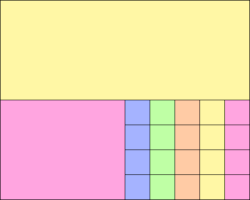下面我们就来解决以上问题。

1. 把矩形块放在固定大小的框内

假设有一个固定大小的矩形框，比如1024x768，我们怎么把矩形块装在里面？答案：使用二叉树

首先在左上角放置第一个（最大的）块，然后将该矩形框剩余的空白区域分割成两个较小的矩形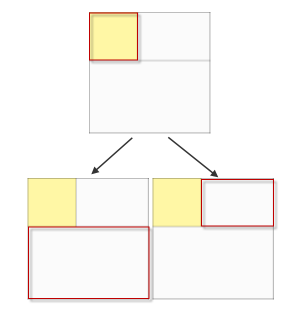以二叉树的形式递归地进行处理，最后得到一个填充的图像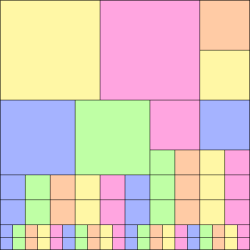程序实现非常简单。假设输入矩形已经按从大到小排序。

Packer = function(w, h) {
this.root = { x: 0, y: 0, w: w, h: h };
};

Packer.prototype = {

fit: function(blocks) {
var n, node, block;
for (n = 0; n < blocks.length; n++) {
block = blocks[n];
if (node = this.findNode(this.root, block.w, block.h))
block.fit = this.splitNode(node, block.w, block.h);
}
},

findNode: function(root, w, h) {
if (root.used)
return this.findNode(root.right, w, h) || this.findNode(root.down, w, h);
else if ((w <= root.w) && (h <= root.h))
return root;
else
return null;
},

splitNode: function(node, w, h) {
node.used = true;
node.down  = { x: node.x,     y: node.y + h, w: node.w,     h: node.h - h };
node.right = { x: node.x + w, y: node.y,     w: node.w - w, h: h          };
return node;
}

}

2. 选择最小宽度和高度

我们现在可以使用一棵二叉树来将矩形块放入一个固定大小的矩形。但是我们应该选择多大的尺寸来确保所有的矩形块都能以最优的方式放置

我考虑了很多启发式方法。其中一个例子是取平均宽度和平均高度，然后分别乘以sqrt（n），以生成一个正方形。n是矩形块的数量。因为使用了平均值，生成矩形块矩形框可能太大也可能太小。

那么有没有别的方法呢？下面来看我们提出的方法。

3. 将矩形块填充进一个不断增长的矩形框

我们不是试图猜测矩形框的最优宽度和高度,我们可以先建立一个小的目标：能容下第一个矩形块。然后在没有足够的空间来容纳下一个块的时候，扩充矩形框。

先来看下没有足够的空间来容纳下一矩形块的情况：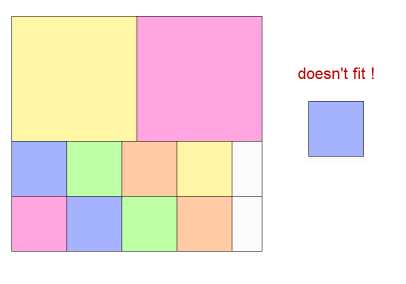这时我们有两种选择，我们可以让矩形框向下生长，或者向右生长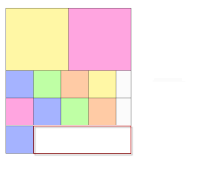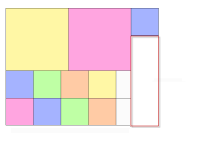这可以通过在原始程序中添加几行代码来实现：

fit: function(blocks) {
var n, node, block;
+     this.root = { x: 0, y: 0, w: blocks.w, h: blocks.h };
for (n = 0; n < blocks.length; n++) {
block = blocks[n];
if (node = this.findNode(this.root, block.w, block.h))
block.fit = this.splitNode(node, block.w, block.h);
+       else
+         block.fit = this.growNode(block.w, block.h);
}
},

• 确保根节点被初始化为与第一个矩形块相同的大小
• 每当findNode返回null时，调用一个新的方法grownode扩充矩形框

实际上，实现grownode方法时需要一些条件判断：

growNode: function(w, h) {

1    var canGrowDown  = (w <= this.root.w);
1    var canGrowRight = (h <= this.root.h);

2    var shouldGrowRight = canGrowRight && (this.root.h >= (this.root.w + w)); // attempt to keep square-ish by growing right when height is much greater than width
2    var shouldGrowDown  = canGrowDown  && (this.root.w >= (this.root.h + h)); // attempt to keep square-ish by growing down  when width  is much greater than height

if (shouldGrowRight)
return this.growRight(w, h);
else if (shouldGrowDown)
return this.growDown(w, h);
else if (canGrowRight)
return this.growRight(w, h);
else if (canGrowDown)
return this.growDown(w, h);
else
return null; // need to ensure sensible root starting size to avoid this happening
},

几点注意事项：

• 如果矩形块比框宽，我们就不能支持矩形框向下生长
• 如果矩形块比框高，我们就不能支持矩形框向右生长

这对算法有相当大的影响。如果一个块比矩形框的宽和高都大，那么我们就不能生长了！解决方案是确保我们的块首先被排序，这样所有后续的块都至少有一个边缘比矩形框小。

这并不是说我们不能支持这个,但会添加额外的复杂性。

• 如果框的高度大于它的宽度再加上块的宽度，那么为了保持近似方形，框向右生长
• 如果框的宽度大于它的高度再加上块的高度，那么为了保持近似方形，框向下生长

这就阻止了我们不断地向右生长并创建一个水平的长条。这也阻止了我们不断的向下生长并创造一个狭窄的垂直地带。它的结果近似于正方形。

4.对矩形块排序

块被放入框的顺序对结果有很大的影响。让我们先看看专家们怎么说：

理论和实证结果表明，‘first fit decreasing’是最好的启发式方法。按照从大到小的顺序排列对象，这样最大的对象第一放入，最小的最后一个放。将每个对象依次地插入到第一个有空间可以容纳它的箱子里。

这里的大小是什么意思？宽度?高度？面积吗?

通过从各种排序算法中进行选择

宽度
高度
面积
maxside——（宽度，高度）
随机-随机化顺序

每一种主要排序也有二级（有时是第三级）排序标准，以避免在其他情况下是相等的。

结果表明，maxside几乎总是最好的选择。意思是，结果大致是正方形的（不是长而细的），并且有最少的空白。展开全文装箱问题
• 该项目是我们工程学院尼斯索菲亚理工学院算法课程的作业。 问题与装箱有关：我们有尺寸相同的容器和各种尺寸的箱子。 目标是使用尽可能少的容器来装满所有的盒子。 他们 == 方法== 我们的方法是对所有高度递减的框...JavaScript
• 装箱问题遗传算法MATLAB实现.doc，这份文档介绍了装箱问题遗传算法MATLAB实现，装箱问题遗传算法MATLAB实现.docmatlab
• 此演示需要HaxeFlixel和装箱的haxelib，因此请首先安装这些： haxelib install flixel haxelib install bin-packing 点击屏幕底部的按钮以测试打包算法。 矩形在添加时会编号，翻转后的矩形会用字母“ F”标记。 ...
• 网线吸收和端口占用分析驱动的FPGA装箱算法.pdf
• 有一些物品，需要将这些物品装到箱子中，求装箱情况，那么我们应该思考如何装箱装箱时要遵循什么样的准则。算法
• SER-Tvpack：基于软错误率评估的SRAM型FPGA的装箱算法.pdf
• 健身器管材的宽高比等于3，并且要求焊缝在角部；长边与短边的连接圆角亦不相等，轧制难度较大。介绍了矩形健身器管焊缝在角部的斜轧轧制工艺及其孔型设计方法，在分析了轧制要求及难点的基础上，给出了孔型设计实施...
• 有160个弹簧，20个箱子，每个箱子可以装8个弹簧，每个箱子放两排。要将这160个弹簧放到箱子里。要求： 每个箱子第一排1—4号i弹簧高度差不超过1mm。第二排5—8高度差不超过1mm，1-8之间的高度差不超过2mm。...
• 承接上一篇推送，今天继续来看看论文 Random Features for Large-Scale Kernel Machines 中提出的第二种随机特征构造方法，姑且叫做随机装箱特征（Random Binnin Features）吧。Random Binning Features第二种特征...

承接上一篇推送，今天继续来看看论文 Random Features for Large-Scale Kernel Machines 中提出的第二种随机特征构造方法，姑且叫做随机装箱特征（Random Binnin Features）吧。

Random Binning Features

第二种特征特征提取方法，有着非常有趣的 Idea。用随机的分辨率和平移量，将数据所在的空间等分成小块，然后记录数据点在哪些小块当中。重复这个操作若干次，看看 2 个数据点被划分到同一个小块区域的频率是多少，用这个频率来近似这 2 个数据点的核函数值（核内积）。直观的来说，当 2 个数据点靠的越近的时候，它们被分到同一个小块区域的频率会越大，这样按上面的 Idea 所逼近的核函数值也应该越大。这是符合许多反应亲密度的核函数的特点。

这个想法也可以用映射的观点来刻画。 令 $$z(x)$$ 是数据点 $$x$$ 所落区域的二进制编号（比如 01011 这样），这样就定义了一个映射 $$z:R^d\to \{0,1\}^D$$，其中 $$D$$ 是编号的位数。 那么逻辑与运算 $$z(x)\&z(y):=z(x)z(y)$$ 的结果为 1 则表示数据点 $$x$$ 和 $$y$$ 落在了同一个区域中，为 0 则表示不在一个区域中。比方说，我们用不同的分辨率和平移量对空间做了 $$P$$ 次分割，对应的有编号映射 $$z_1,\cdots,z_P$$。这样，数据点 $$x$$ 和 $$y$$ 落在同一个区域中的频率就是：

1Pp=1Pzp(x)zp(y):=z(x)Tz(y)k(x,y)

其中 $$z(x)=\frac{1}{\sqrt{P}}[z_1(x) \cdots z_P(x)]$$，就是我们要找的特征映射。

带着这个 Idea，问题的重心就落在了如何随机的选取空间分割的分辨率和平移量，使得上面的近似能够尽可能精确。

首先我们要利用概率论知识来对整个分割空间的操作进行刻画，然后考察上述近似的精确度，并设法提高。一般思路是，确定分割区域的分辨率和平移量应该服从什么分布，才能使得频率 $$z(x)^Tz(y)$$ 是 $$k(x,y)$$ 的无偏估计，然后刻画分割次数 $$P$$ 对近似的精确度有何影响，比如估计随着 $$P$$ 增大，$$z(x)^Tz(y)$$ 收敛到 $$k(x,y)$$ 的速度（如果收敛的话）。

先考虑 1 维的情形。假设有一个核函数 $$k(x,y)$$。给定任意 2 个实数轴上的点 $$x,y\in R$$。我们把实数轴用随机选取的间隔 $$\delta$$ 等分成一系列区间，设 $$p(\delta),\delta>0$$ 是 $$\delta$$ 服从的分布。然后再从 $$[0,\delta]$$ 的均匀分布中随机取 $$u$$ 作为分割区间的偏移量，最后将整条实数轴均分成形如 $$[u+k\delta,u+(k+1)\delta),n\in Z$$ 的一系列区间。现在，为了让 $$z(x)z(y)\approx k(x,y)$$，当然首先希望 $$z(x)z(y)$$ 是 $$k(x,y)$$ 的无偏估计，就是说，我们希望：

k(x,y)=Eδ,u[z(x)z(y)]

所以问题就集中在，怎么确定分布 $$p(\delta)$$ 使得上式成立。考虑到在分割中，我们是先取定 $$\delta$$，再取定 $$u$$ 的，于是想到把 $$\delta$$ 作为条件，利用条件期望定义，得到：
Eδ,u[z(x)z(y)]=Eδ[Eu[z(x)z(y)|δ]]=0Eu[z(x)z(y)|δ]p(δ)dδ

回忆 $$z(x)z(y)$$ 的含义：
z(x)z(y)={10x,y,

于是可以计算：
Eu[z(x)z(y)|δ]=Pru[z(x)z(y)=1|δ]

接下来再计算上式右边，也就是 $$x,y$$ 两个点落在同一个区间的概率。当 $$|x-y|>\delta$$ 的时候，2 个点无论如何都不可能在同一个区间内，因此这时它们落在同一区间内的概率是 0；而当 $$|x-y|\leq\delta$$ 时，由几何知识知道，给定的 2 点落在同一个区间的概率是 $$1-\frac{|x-y|}{\delta}$$。因此，综合起来有：
Pru[z(x)z(y)=1|δ]=max(0,1|xy|δ):=k^(x,y;δ)

这样，我们就得到了确定分布 $$p(\delta)$$ 的一个积分方程：

k(x,y)=0k^(x,y;δ)p(δ)dδ=|xy|(1|xy|δ)p(δ)dδ

这时，就要对核函数的形状做一些约束了。假设核函数只和数据点的 $$L_1$$ 距离有关，即有这样的形状：
k(x,y)=k(|xy|)

这样，如果记 $$\Delta=|x-y|$$，上述方程改写成：
k(Δ)=Δ(1Δδ)p(δ)dδ

两边对 $$\Delta$$ 求 2 次导数，就可以得到：
Δ2kΔ2=p(Δ)

至此，就得到了确定分布 $$p$$ 的公式。并且，由于 $$p$$ 是一个分布函数，上式成立，自然要求核函数是凸的，这样它的二阶导数才会大于 0。比如 Gauss 核函数 $$e^{-|x-y|^2}$$ 就不是这样的函数，也就是说，这次讨论的随机装箱特征不可能使用在 Gauss 核函数上面。但是 Laplace 核函数 $$e^{-|x-y|}$$ 就完全符合上面所有的要求，可以说随机装箱特征完全就是为 Laplace 核函数量身定做的。比如，Laplace 核函数对应的分布 $$p$$ 恰好是 Gamma 分布函数 $$\delta e^{-\delta}$$。

接下来，就是重复做 $$P$$ 次上面的分割，每次都随机的从分布 $$p$$ 取不同的分辨率 $$\delta$$，从区间 $$[0,\delta]$$ 随机的取偏移量 $$u$$，得到一系列编码映射 $$z_1,\cdots,z_P$$。因为每个 $$z_p(x)z_p(y)$$ 都是核函数 $$k(x,y)$$ 的无偏估计，所以统计任意 2 点落在同一区间的频率：

1Pp=1Pzp(x)zp(y):=z(x)Tz(y)k(x,y)

也是核函数的一个无偏估计，而且方差更小。

到这里，我们就已经得到了 1 维情形的随机装箱特征算法，更高维的讨论是类似的，论文里面有相关讨论，这里就不费口舌了。我们把 1 维情形的算法整理如下：

算法 随机装箱特征
前提：数据空间 1 维。核函数 $$k(x,y)$$ 有形状 $$k(|x-y|)=k(\delta)$$，而且用下式构造的函数

p(δ)=δ2kδ2,δ>0

是一个概率密度函数。
效果：得到随机特征映射 $$z(x)$$ 可以使得 $$z(x)^Tz(y)\approx k(|x-y|)$$。
for $$m=1,\cdots,P$$
从分布 $$p$$ 中随机选取分辨率 $$\delta_m$$，从区间 $$[0,\delta_m]$$ 内随机选取偏移量 $$u_m$$，把实数轴等分成一系列区间。
把有数据点下落的区间用二进制编码，用 $$z_p(x)$$ 表示数据 $$x$$ 下落的区间编号。
end for
令 $$z(x)=\frac{1}{\sqrt{P}}[z_1(x) \cdots z_P(x)]$$ 得所求。

可能要提下的是，论文里面没有提到用随机装箱特征的话，evaluation 里面 $$w^Tz(x)=\frac{1}{P}\sum_p w_pz_p(x)$$ 里面权重的每一个分量是什么，那么为了统一运算，可以姑且认为也是一个二进制串。

最后，论文还讨论了随机装箱特征逼近核函数的收敛速度，这一段是很体现作者数学功力的。它的思路是从概率测度的意义上探讨算法随着分割次数 $$P$$ 增大，逼近过程的收敛速度。结论是，逼近达到指定精确度的概率随着 $$P$$ 增大，成指数增长到 1。有需要的话，笔者可能会专门花一篇文章来学习作者的这些技巧。

后记
总体而言，整篇论文的奇思妙想非常多，阅读过程也很愉快。但是可以看到，随机装箱特征适用的核函数是有限的，相比较起来，随机 Fourier 特征的适用范围更广一些。但是随机装箱特征也是有用武之地的，比如论文的实证部分提到的，一些分类问题数据集的分割平面高度不光滑，这时候随机 Fourier 特征的效果就远不如随机装箱特征。
这篇论文给我们的启示是，可以多用概率分布来刻画带有随机性的操作，然后借用概率论和数理统计的知识对问题进行建模和解决。另外，论文在推导随机 Fourier 特征时提到的那个调和分析的定理，也启发我们，看到一些概率密度或者测度的相关定理，也应该反方向的思考是否可以由此开发出对应的随机操作。

展开全文人工智能 机器学习 随机特征
• 该项目包含使用遗传算法解决的装箱问题的解决方案。 该项目中的代码是作为2007年南里奥格兰德州联邦大学（UFRGS-巴西）组合优化课程中问题的解决方案而创建的。开源软件
• 贪心算法装箱问题，使用c语言来实现的，贪心算法装箱问题，使用c语言来实现的，贪心
• 装箱算法简单描述如下： { 输入箱子的容积； 输入物品种数n； 按体积从大到小顺序，输入各物品的体积； 预置已用箱子链为空； 预置已用箱子计数器box_count为0； for (i=0;i 从已用的第一只箱子开始顺序寻找能放入...
• 本篇文章再次介绍了C#中的装箱与拆箱，这次们看下使用泛型和不使用泛型引发装箱拆箱的情况
• 三维装箱遗传算法matlab程序

千次阅读 2021-04-22 13:48:13
三维装箱遗传算法matlab程序基于遗传模拟退火算法的三维装箱问题研究从计算复杂性理论来讲,装箱问题是一个 NP 难题,很难精确求解。目前的求解方法主要是一些近似算法, 如 NF(NextFit)近似算法、FF(FirstFit)近似算法...
• php实现装箱算法贪婪法是一种不追求最优解，只希望得到较为满意解的方法。贪婪法一般可以快速得到满意的解，因为它省去了为找最优解要穷尽所有可能而必须耗费的大量时间。贪婪法常以当前情况为基础作最优选择，而不...php装箱...